Topic 5 min read# Ohmic drop correction: a means of improving measurement accuracy

Latest updated: August 26, 2021

Introduction

The ohmic drop in electrochemistry indicates the potential induced by the current flow through the electrolyte (with an inherent resistance) or any other homogenously resistive components such as surface films or connectors. It can have a non-negligible influence on different types of measurements in electrochemistry when using potentiostats or battery cyclers.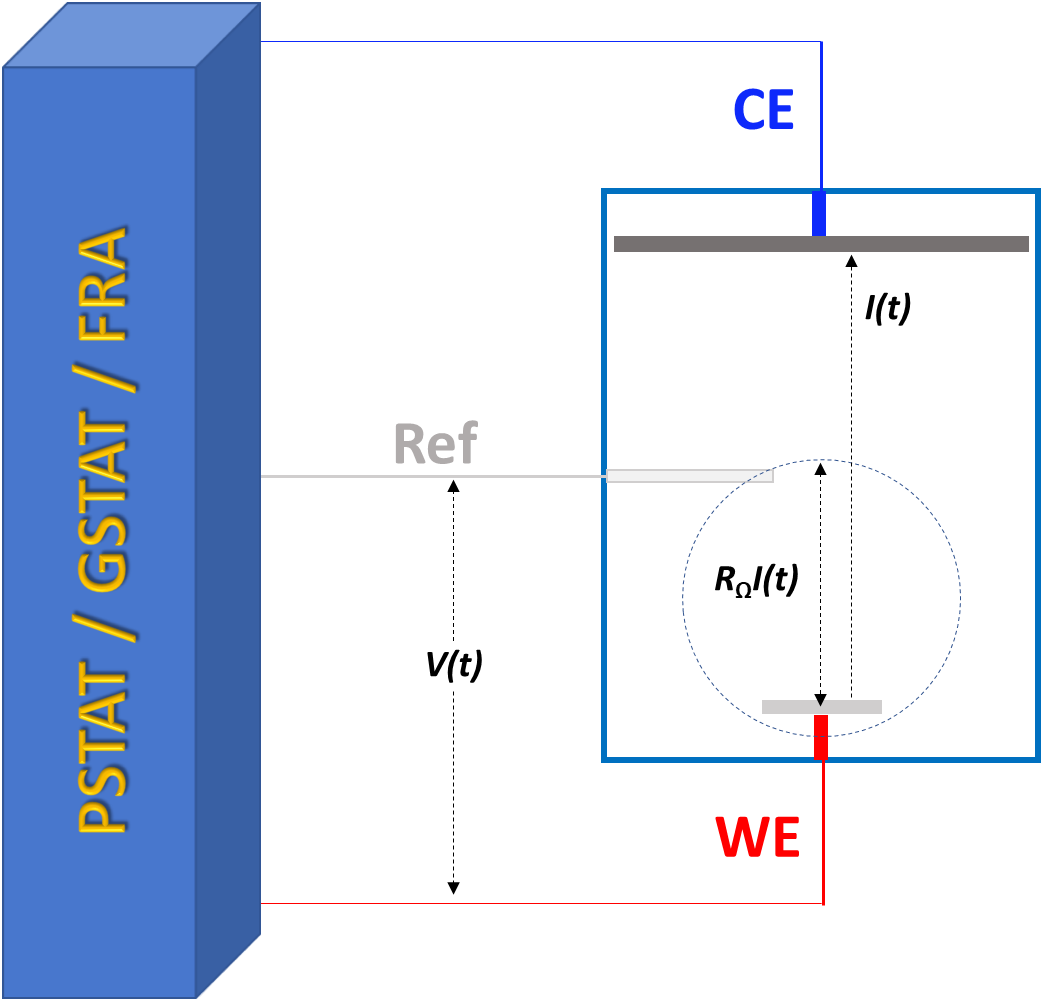Figure 1: Ohmic drop in the standard three-electrode set-up.

The ohmic drop is equal to $R_\Omega I(t)$ in the equation below where $V(t)$ is the potential “seen” by the electrode and $E(t)$ the desired potential:

$$V(t)=E(t)+R_\Omega I(t) \tag{1}$$

Proper cell design can significantly reduce the ohmic potential drop in the solution prior to the measurement, for example, by placing the reference electrode as close as possible to the working electrode. However, in some cases, for example low conductive electrolytes and/or large-scale electrochemical systems, it is not possible to remove this contribution from the measured potential and supplementary techniques are required to determine and correct the ohmic drop.

Ohmic drop can have an influence on the shape of the measured curve and become a source of error during the analysis of the results obtained. Examples of electrochemical measurements affected by the ohmic drop are shown in Figures 2 and 3. Measurements were recorded with a VMP3e multichannel potentiostat/galvanostat: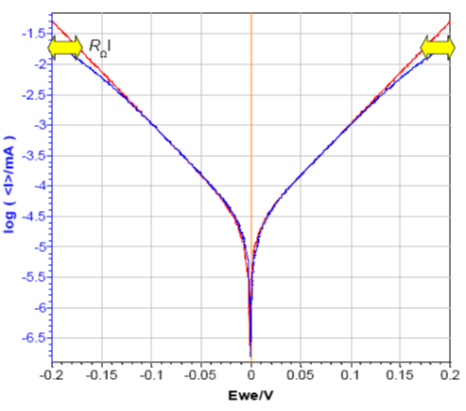Figure 2: Tafel plot of circuit #2 of TestBox-3. No resistance (red) and
Ω (blue) in series with the WE.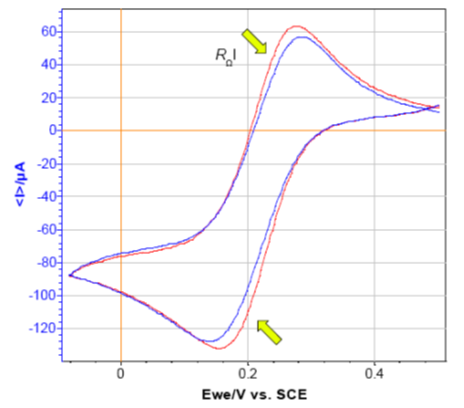Figure 3: CV curves of [(Fe(CN)63-] (0.6 mM) + KCl (0.1 M). No resistance (red) and resistance
of 100
Ω (blue) in series with the WE (RΩI ≈ 10 mV at anodic current peak).

Various techniques are available in EC-Lab®, the control and analysis software for BioLogic potentiostats, to determine the value of the resistance causing the ohmic drop: current interrupt and EIS (see AN #28).

Electrochemical Impedance Spectroscopy is well-suited to obtain the right value of the ohmic drop whatever the kinetics of the system. Fitting the results with an equivalent circuit allows users to obtain an accurate value of the ohmic resistance (R1 in Figure 4).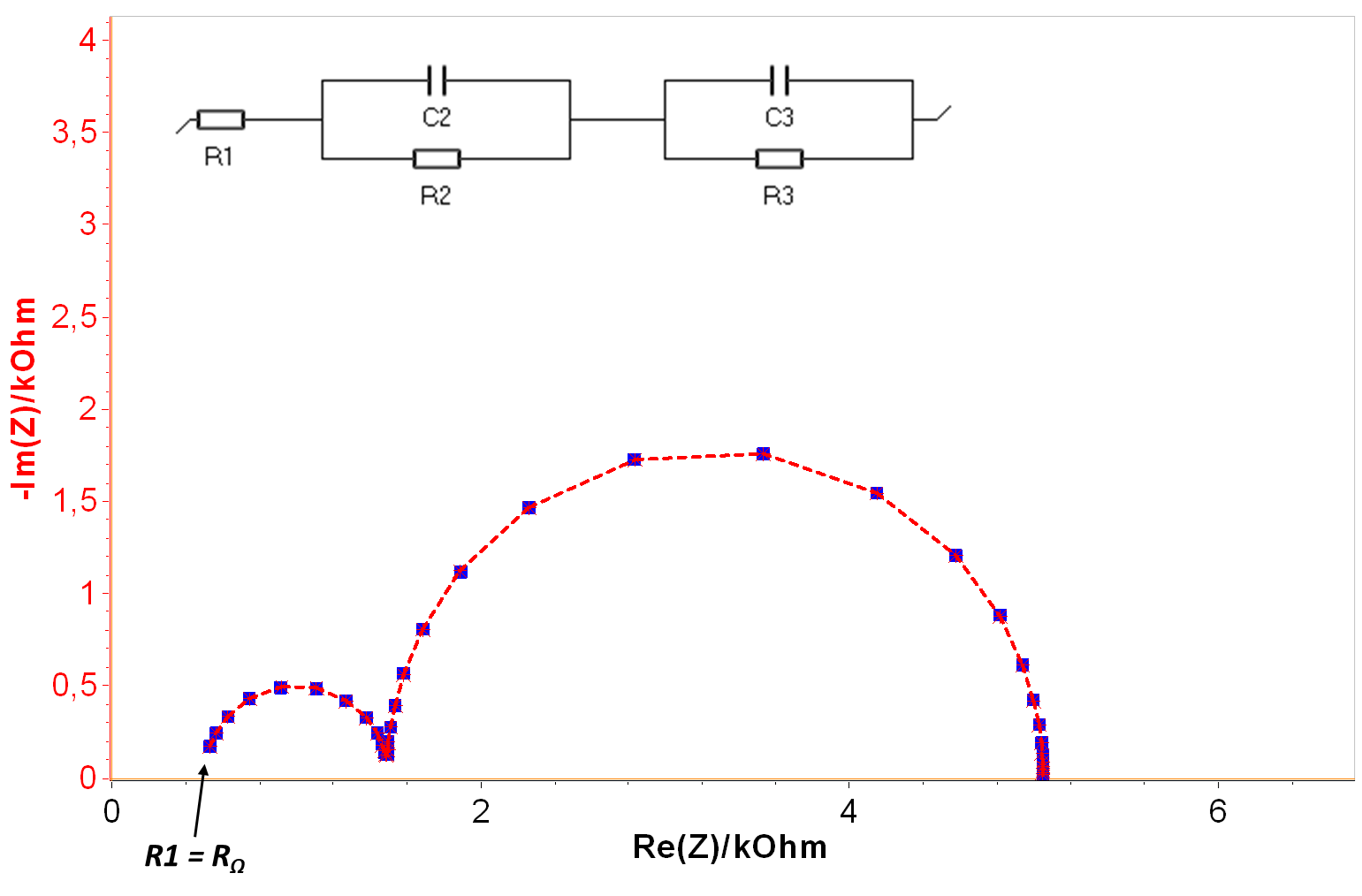Figure 4: Nyquist diagram of the impedance of circuit #1-Test Box 3 (blue),
resulting fit obtained with ZFit for the equivalent circuit (red).

Moreover, beyond the determination of the ohmic drop, EC-Lab® and EC-Lab® Express software makes it possible to compensate its influence, simply by linking an ohmic drop determination technique before the technique of interest (Figs. 5 and 6).

The value of the resistance to be compensated can be entered manually (MIR, Fig. 5), measured by impedance at one frequency (ZIR, Fig. 6), or by the Current Interrupt technique (CI).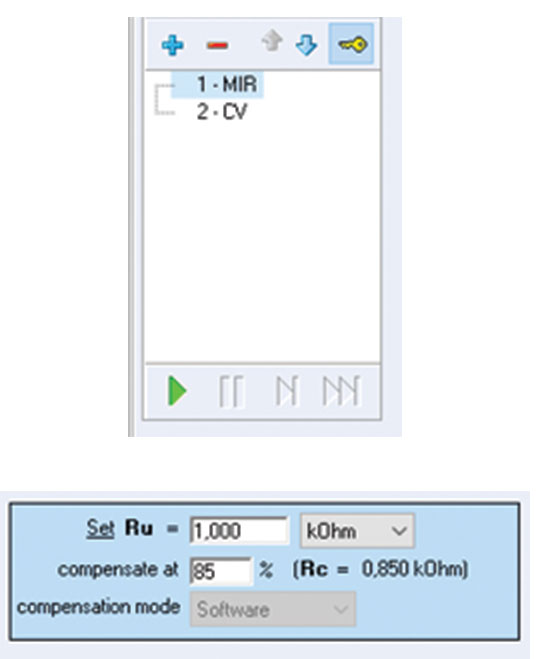Figure 5: Ohmic drop compensation in linked experiments and
parameters settings window of MIR experiment in EC-Lab
®.

In ZIR, $R_\Omega$ is calculated as follows:

$$R_\Omega=\mathrm{Re}Z(f_\mathrm{ZIR})\tag{2}$$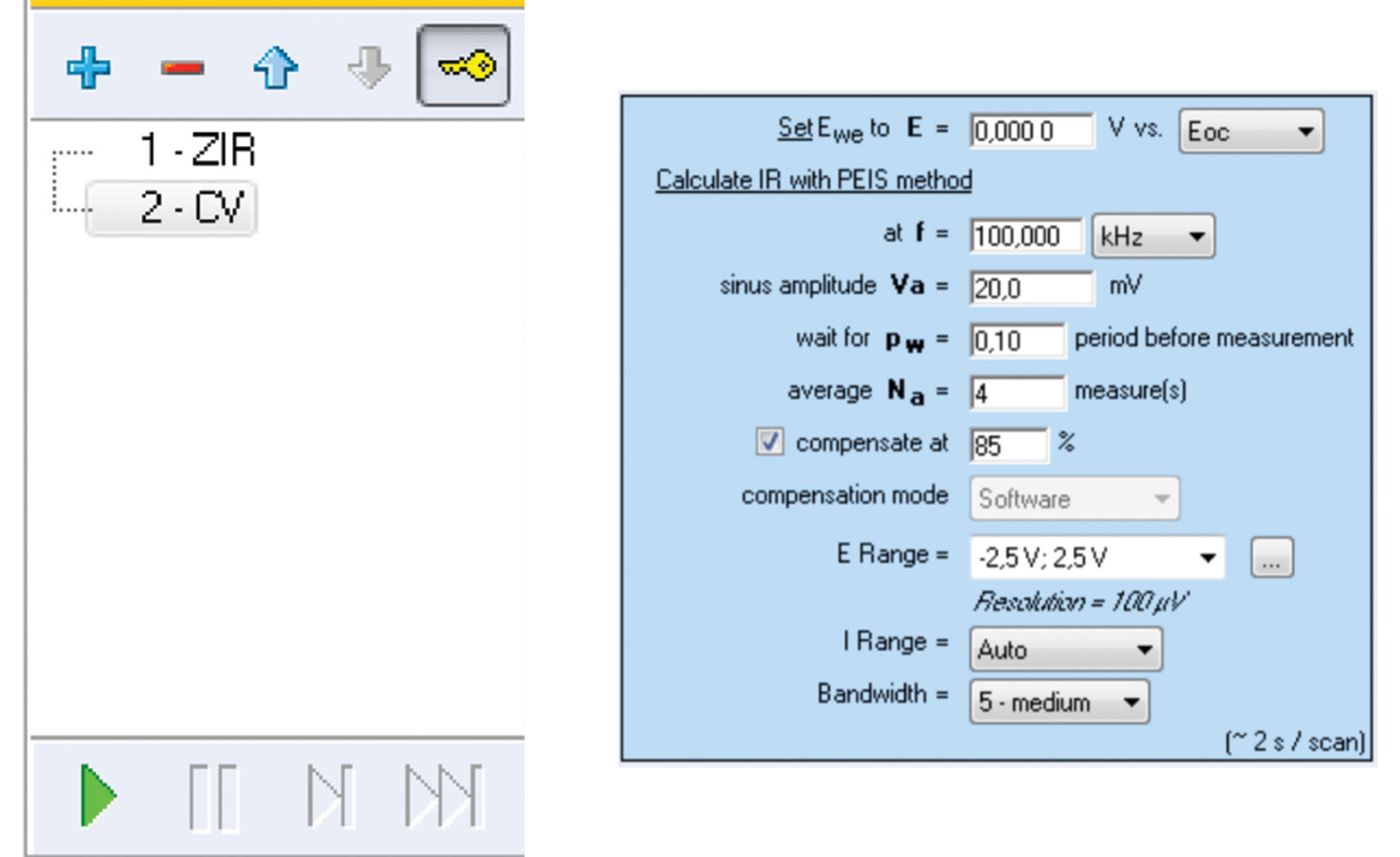Figure 6: Ohmic drop compensation in linked experiments and parameters
settings window of ZIR experiment in EC-Lab
®.

The ZIR tool allows the user to obtain an immediate resistance value of the ohmic drop without the need to know the electrical equivalent circuit. By default, this measurement is performed for 100 kHz, but users can change this value according to the studied system.

Please note that hardware compensation will not work if the resistance value to be compensated is larger than the shunt used to measure the current. For software compensation, there is no limitation. For example, if the resistance to be compensated is 150 Ω and the measured current is 8 mA, then the current range used is 10 mA, which corresponds to 100 Ω, the ohmic drop cannot be compensated.

Furthermore, if the system contains an inductive behavior in the high-frequency range, a preliminary impedance experiment and analysis with ZFit must be carried out in order to determine a reasonable frequency for ZIR (see AN #29).

AN #27 “Ohmic Drop, Part I: Effect on measurements”

AN #28 “Ohmic Drop. II – Introduction to Ohmic Drop measurement techniques.”

ohmic drop potentiostat ohmic potential drop EIS Electrochemical Impedance Spectroscopy Current Interrupt technique Ohmic drop compensation

## Related products

Work smarter. Not harder.

Tech-tips, theory, latest functionality, new products & more.

No thanks!